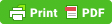# IMPORTANT SCIENTIFIC INSTRUMENT AND THEIR USES THAT ASK IN EXAMINATION

1. Altimeter :- It measures altitudes and is used in aircrafts.
2. Ammeter :- It measures strength of electric current.
3. Audiometer :- It measures intensity of sound.
4. Barometer :- It measures atmospheric pressure.
5. Binocular :- It is used to view distant objects.
6. Calorimeter :- It measures quantity of heat.
7. Cardiogram :- It trace movement of heart, recorded on a cardiograph.
8. Dynamo :- It converts mechanical energy into electrical energy.
9. Fathometer :- It measures the depth of the ocean.
10. Galvanometer :- It measures the electric current of low magnitude.
11. Hydrometer :- It measures the specific gravity of liquids.
12. Hygrometer :- It measures humidity in air.
13. Lacometer :- It determines the purity of milk.
14. Manometer :- It measures the pressures of the gases.
15. Odometer :- It is an instrument by which the distance covered by wheeled vehicles is measured.
16. Phonograph :- It is used for producing sounds.
17. Photometer :- It compares the luminous intensity of the source of light.
18. Periscope :- It is used in submaines to view objects above sea level.
19. Radar :- It is used for detecting the direction and range of an approaching plane by means of radio microwaves.
20. Seismograph :- It measures intensity of earthquake.
21. Salinometer :- It determine salinity of solution.
22. Sonometer :- It measures frequency of a tunning fork.
23. Speedometer :- It is an instrument placed in a vehical to record its speed.
24. Sphygmomanometer :- It is used to measure blood pressure.
25. Stethoscope :- It is an instrument which is used by the doctors to hear and analyse the heart and lung sounds.
26. Thermometer :- This instrument is used for the measurement of temprature.
27. Thermostat :- It regulates the temprature at a particular point.
28. Voltmeter :- It measures the electric potential difference between two points.

#### Biology other lectures:-